# Raoult's law

Raoult's law states that the vapour pressure of an ideal solution of$N$ components is: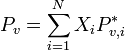$P_v = \sum_{i=1}^{N} X_i P^*_{v,i}$
where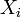$X_i$ is the molar fraction of component$i$, and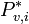$P^*_{v,i}$ is the vapour pressure of pure$i$. More generally, Raoult's law describes the partial pressure of component$A$ in the vapour coexisting with a liquid mixture as: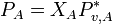$P_A = X_A P^*_{v,A}$.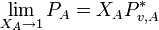$\lim_{X_A \rightarrow 1} P_A = X_A P^*_{v,A}$.